UPSC  >  Practice Test: Ratio & Proportion- 2

# Practice Test: Ratio & Proportion- 2 - UPSC

Test Description

## 10 Questions MCQ Test CSAT Preparation - Practice Test: Ratio & Proportion- 2

Practice Test: Ratio & Proportion- 2 for UPSC 2023 is part of CSAT Preparation preparation. The Practice Test: Ratio & Proportion- 2 questions and answers have been prepared according to the UPSC exam syllabus.The Practice Test: Ratio & Proportion- 2 MCQs are made for UPSC 2023 Exam. Find important definitions, questions, notes, meanings, examples, exercises, MCQs and online tests for Practice Test: Ratio & Proportion- 2 below.
Solutions of Practice Test: Ratio & Proportion- 2 questions in English are available as part of our CSAT Preparation for UPSC & Practice Test: Ratio & Proportion- 2 solutions in Hindi for CSAT Preparation course. Download more important topics, notes, lectures and mock test series for UPSC Exam by signing up for free. Attempt Practice Test: Ratio & Proportion- 2 | 10 questions in 20 minutes | Mock test for UPSC preparation | Free important questions MCQ to study CSAT Preparation for UPSC Exam | Download free PDF with solutions
 1 Crore+ students have signed up on EduRev. Have you?
Practice Test: Ratio & Proportion- 2 - Question 1

### The ratio of the number of boys and girls in a college is 7 : 8. If the percentage increase in the number of boys and girls be 20% and 10% respectively, what will be the new ratio?

Detailed Solution for Practice Test: Ratio & Proportion- 2 - Question 1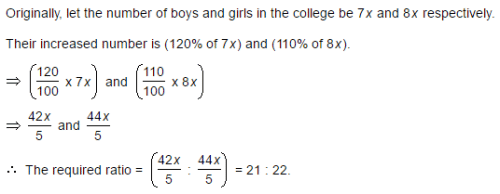Practice Test: Ratio & Proportion- 2 - Question 2

### Salaries of Ravi and Sumit are in the ratio 2 : 3. If the 'salary of each' one of them is increased by Rs. 4000, the new ratio becomes 40 : 57. What is Sumit's present salary?

Detailed Solution for Practice Test: Ratio & Proportion- 2 - Question 2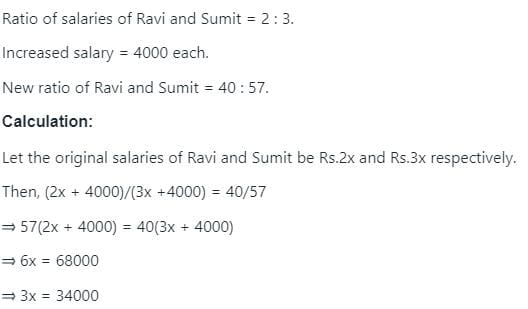∴ Sumit's present salary = (3x + 4000) = Rs.(34000 + 4000) = Rs. 38000.

Practice Test: Ratio & Proportion- 2 - Question 3

### The salaries A, B, C are in the ratio 2 : 3 : 5. If the increments of 15%, 10% and 20% are allowed respectively in their salaries, then what will be new ratio of their salaries?

Detailed Solution for Practice Test: Ratio & Proportion- 2 - Question 3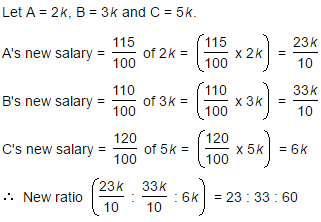Practice Test: Ratio & Proportion- 2 - Question 4

If 40% of a number is equal to two-third of another number, what is the ratio of first number to the second number?

Detailed Solution for Practice Test: Ratio & Proportion- 2 - Question 4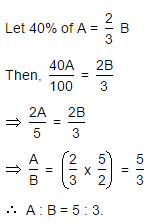Practice Test: Ratio & Proportion- 2 - Question 5

The fourth proportional to 5, 8, 15 is:

Detailed Solution for Practice Test: Ratio & Proportion- 2 - Question 5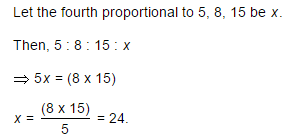Practice Test: Ratio & Proportion- 2 - Question 6

In a bag, there are coins of 25 p, 10 p and 5 p in the ratio of 1 : 2 : 3. If there is Rs. 30 in all, how many 5 p coins are there?

Detailed Solution for Practice Test: Ratio & Proportion- 2 - Question 6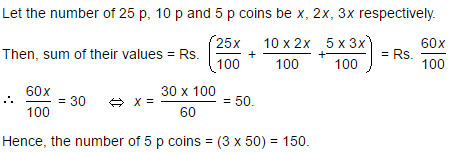Practice Test: Ratio & Proportion- 2 - Question 7

Two number are in the ratio 3 : 5. If 9 is subtracted from each number, the new numbers are in the ratio 12 : 23. The smaller number is:

Detailed Solution for Practice Test: Ratio & Proportion- 2 - Question 7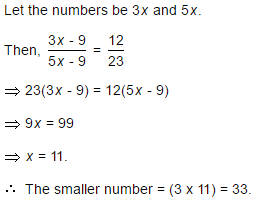Practice Test: Ratio & Proportion- 2 - Question 8

If 0.75 : x :: 5 : 8, then x is equal to:

Detailed Solution for Practice Test: Ratio & Proportion- 2 - Question 8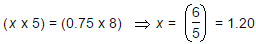Practice Test: Ratio & Proportion- 2 - Question 9

The sum of three numbers is 98. If the ratio of the first to second is 2 :3 and that of the second to the third is 5 : 8, then the second number is:

Detailed Solution for Practice Test: Ratio & Proportion- 2 - Question 9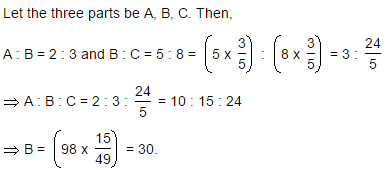Practice Test: Ratio & Proportion- 2 - Question 10

In a garrison of 3600 men, the provisions were sufficient for 20 days at the rate of 1.5 kg per man per day. If x more men joined, the provisions would be sufficient for 12 days at the rate of 2 kg per man per day. Find x.

Detailed Solution for Practice Test: Ratio & Proportion- 2 - Question 10

Let x be the number of new men joined the garrison,
The total quantity of food is = 3600(20) (1.5) kg ----------1
Now the available food will be consumed by (3600 + x) men
(3600 + x) (12) (2) kg  --------------2
1 = 2
Solving both the equations
3600(20) (1.5) = (3600 + x) (12) (2)
108000 = 86400 + 24x
21600 = 24x
X = 900
900 more men joined the garrison.

## CSAT Preparation

197 videos|151 docs|200 tests
Information about Practice Test: Ratio & Proportion- 2 Page
In this test you can find the Exam questions for Practice Test: Ratio & Proportion- 2 solved & explained in the simplest way possible. Besides giving Questions and answers for Practice Test: Ratio & Proportion- 2, EduRev gives you an ample number of Online tests for practice

## CSAT Preparation

197 videos|151 docs|200 tests

### How to Prepare for UPSC

Read our guide to prepare for UPSC which is created by Toppers & the best Teachers(Scan QR code)